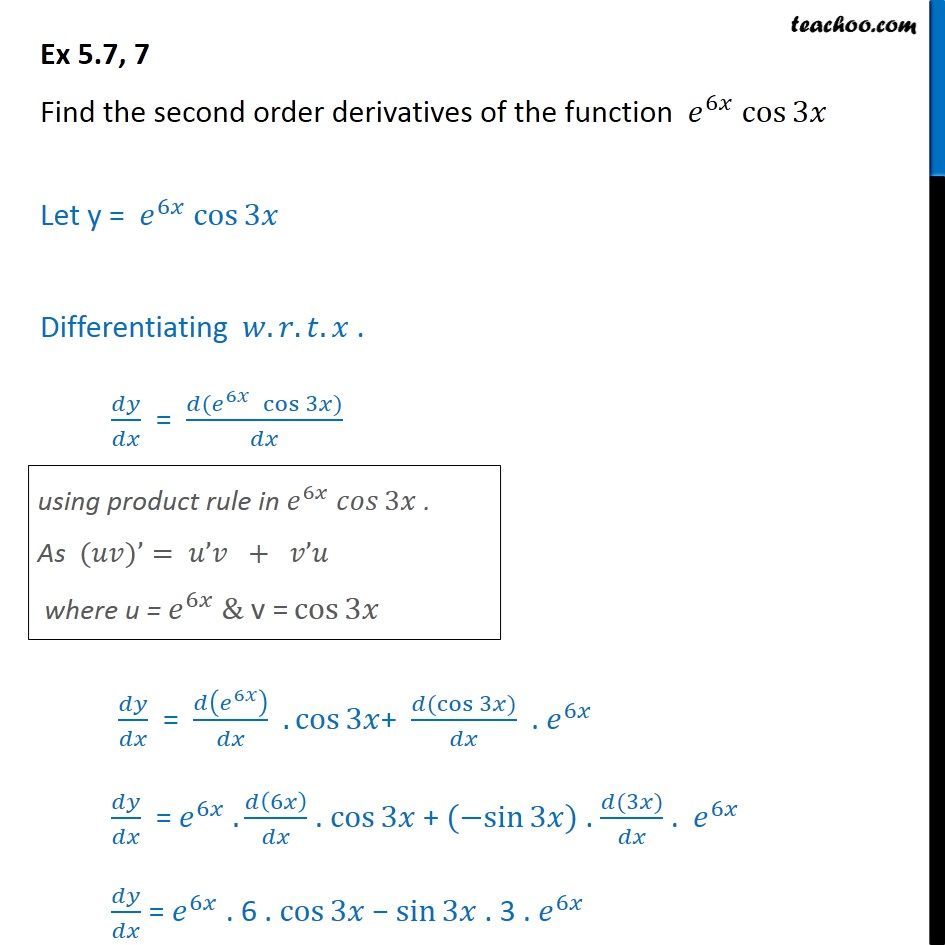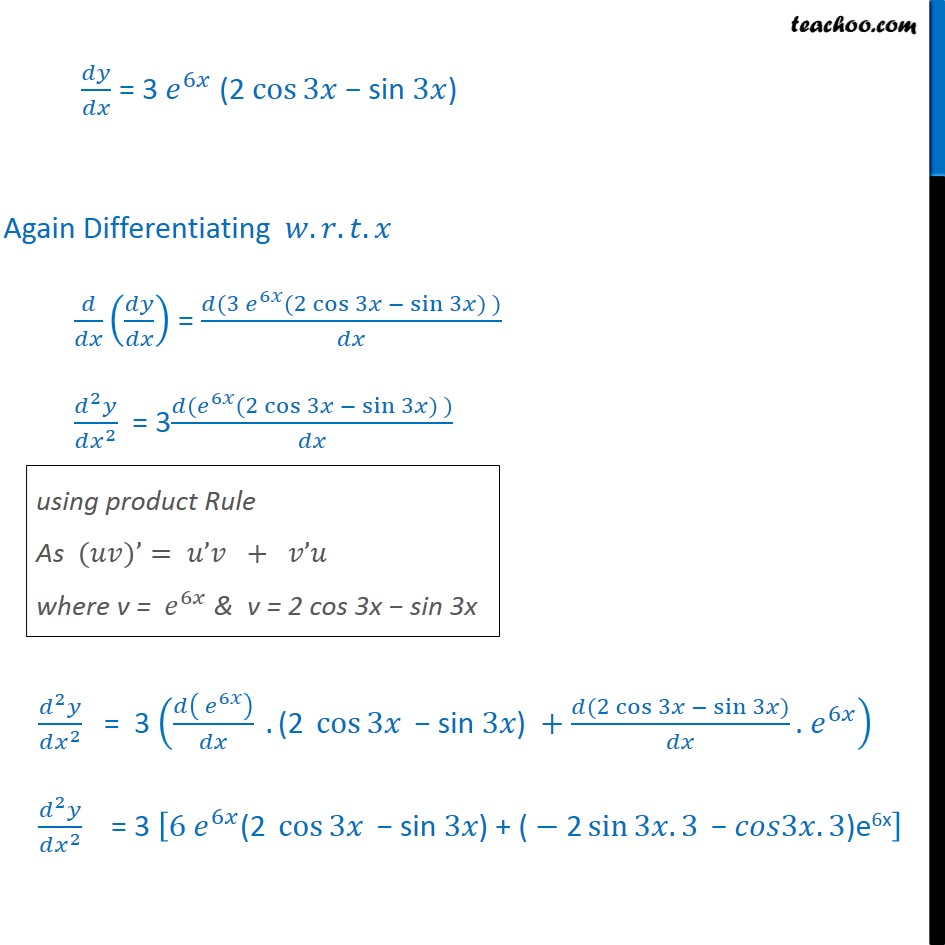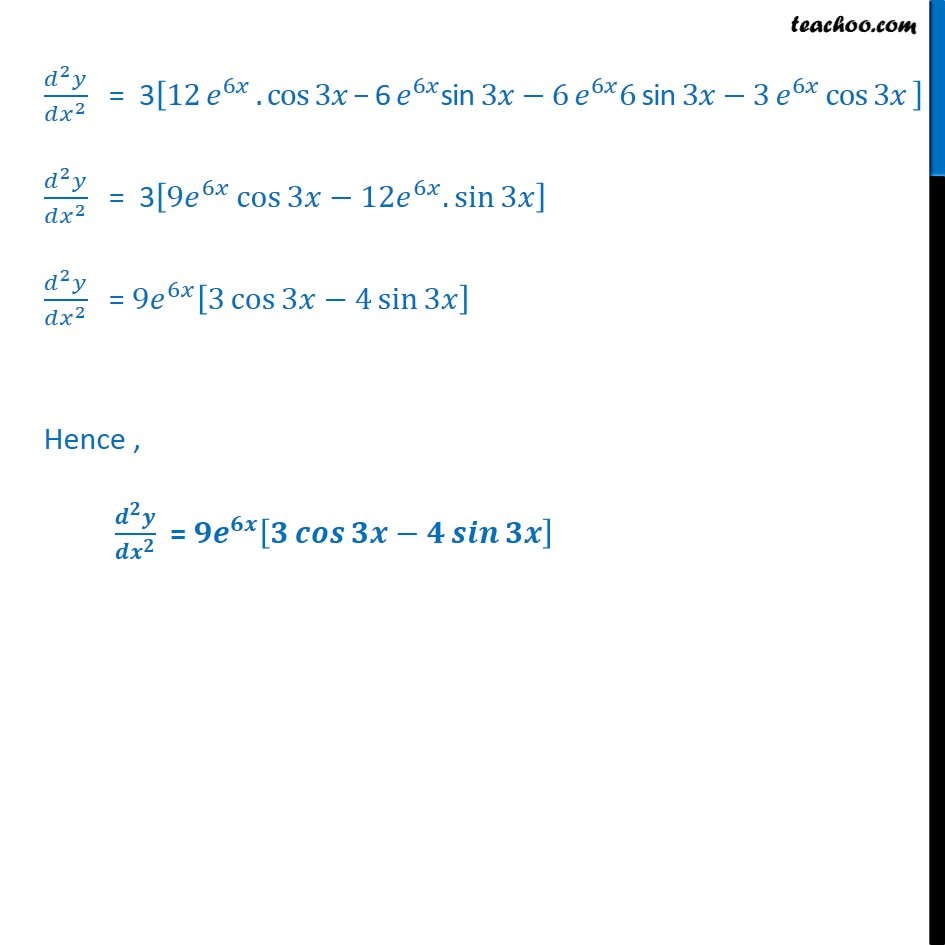1. Chapter 5 Class 12 Continuity and Differentiability
2. Serial order wise
3. Ex 5.7

Transcript

Ex 5.7, 7 Find the second order derivatives of the function 6 cos 3 Let y = 6 cos 3 Differentiating . . . . = ( 6 cos 3 ) = 6 . cos 3 + ( cos 3 ) . 6 = 6 . 6 . cos 3 + ( sin 3 ) . (3 ) . 6 = 6 . 6 . cos 3 sin 3 . 3 . 6 = 3 6 (2 cos 3 sin 3 ) Again Differentiating . . . = (3 6 (2 cos 3 sin 3 ) ) 2 2 = 3 ( 6 (2 cos 3 sin 3 ) ) 2 2 = 3 6 .(2 cos 3 sin 3 ) + (2 cos 3 sin 3 ) . 6 2 2 = 3 6 6 (2 cos 3 sin 3 ) + ( 2 sin 3 .3 3 .3)e6x 2 2 = 3 12 6 .cos 3 6 6 sin 3 6 6 6 sin 3 3 6 cos 3 2 2 = 3 9 6 cos 3 12 6 . sin 3 2 2 = 9 6 3 cos 3 4 sin 3 Hence , =

Ex 5.7